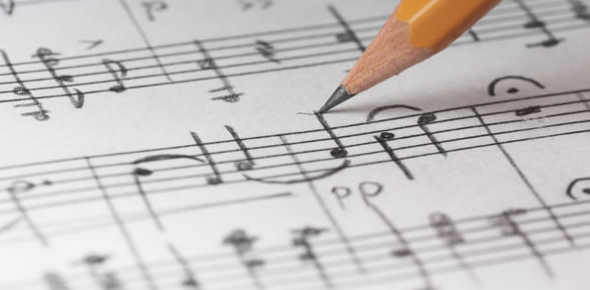# Basic Music Theory Quiz! Trivia

26 Questions | Total Attempts: 484SettingsCreate your own QuizDoes the thought of music theory have you humming a tune in delight? Music theory is a practice that musicians use to appreciate and communicate the language of music. Musical theory reviews the basics of music. It also gives a system to interpret musical arrangements. For example, basic music theory characterizes the elements that create harmony, melody, and cadence. This quiz will get you into the rhythm of basic music theory.

• 1.
Type response below
• 2.
What note is a perfect fifth interval above C?
• A.

D

• B.

E flat

• C.

E

• D.

F

• E.

G

• 3.
What does the lower number in the time signature indicate?
• A.

Number of beats per measure

• B.

Number of measures per barline/staff

• C.

Length of note that is one beat

• D.

Pitch of note that is one beat

• E.

Number of beats in two measures

• 4.
What is the relative minor scale to G major?
• A.

B minor

• B.

C minor

• C.

C sharp minor

• D.

D minor

• E.

E minor

• 5.
Which of the following markings would affect the length of a note?
• A.

Sharp

• B.

Double-sharp

• C.

Flat

• D.

Dot

• E.

None of the above

• 6.
Which of the following is the correct term for a note that is an 8th above another note?
• A.

Relative major

• B.

Perfect

• C.

Octave

• D.

Dominant

• E.

Minor

• 7.
What would the dominant chord be in the key of A major?
• A.

CEG

• B.

EG#B

• C.

FAC

• D.

AC#E

• E.

BDF#

• 8.
How would the chord (with notes from lowest to highest) : C E G B be classified?
• A.

Major 7th

• B.

Minor 6th

• C.

Perfect 5th

• D.

Augmented 7th

• E.

Major 9th

• 9.
How many sharps are in the scale F# (sharp) major?
• A.

2

• B.

3

• C.

4

• D.

5

• E.

6

• 10.
The key signature of Bb (B flat major) has 3 flats
• A.

True

• B.

False

• 11.
The key signature of B (major) has 5 sharps
• A.

True

• B.

False

• 12.
A major 3rd interval is equivalent to two whole steps above the root
• A.

True

• B.

False

• 13.
B minor is the relative minor to Eb major
• A.

True

• B.

False

• 14.
In 4/4 time, which note receives one full beat?
• A.

Sixteenth note

• B.

Half note

• C.

Quarter note

• D.

Whole note

• 15.
What note is a perfect fourth interval above A?
• A.

B

• B.

D

• C.

F#

• D.

Eb

• 16.
What note is a major seventh interval above E?
• A.

C#

• B.

D

• C.

B

• D.

D#

• 17.
The A major chord is the V (5) chord in the key of
• A.

C

• B.

E

• C.

D

• D.

Bb

• 18.
E major in its first inversion would be
• A.

B G# E

• B.

G# B E

• C.

E G B

• D.

A C# E

• 19.
What note is a minor 3rd interval above C?
• A.

Eb

• B.

F

• C.

Db

• D.

A#

• 20.
What does the top number in the time signature indicate?
• A.

How many beats per measure

• B.

How many measures per section

• C.

The length of each note

• D.

The tempo of the song

• 21.
Two sixteenth notes are the equivalent of
• A.

One half note

• B.

One eighth note

• C.

Two quarter notes

• D.

One quarter note

• 22.
If the time signature is 3/4, which note takes one full beat?
• A.

Quater note

• B.

Triplet

• C.

Sixteenth note

• D.

Eighth note

• 23.
If the time signature is 6/8, which note takes one full beat?
• A.

Half note

• B.

Sixteenth note

• C.

Eighth note

• D.

Thirty-second note

• 24.
How would the chord (with notes from lowest to highest) : B D F# A be classified?
• A.

Major 7th

• B.

Perfect 5th

• C.

Minor 7th

• D.

Augmented 7th

• E.

Major 9th

Related TopicsBack to top
×

Wait!
Here's an interesting quiz for you.